The term linear combination is fundamental to linear algebra and will be used throughout this text. A linear combination of a set of vectors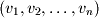can be defined as the addition of these vectors scaled by a corresponding ordered set of scalar coefficients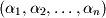: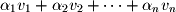For example, let's consider the following 3 vectors: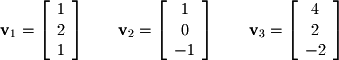In this case,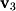is a linear combination of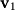and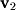. Close inspection shows that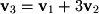: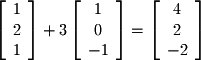This linear combination is illustrated graphically in Figure 2-4 where you can see thatis composed of 1and 3's.
Object could not be loaded.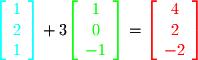Figure 2-4.
Geometry of a Linear Combination
Linear combinations will often be used to define more complex mathematical sets or geometric objects. For example, a line in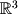is defined as the combination of a starting vector (in this case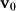) with a direction vector (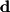) which is scaled by a "free parameter". The term free parameter simply states that the scalar value is free to take on any real value between positive and negative infinity or in interval notation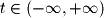. Figure 2-5 illustrates how this linear combination maps out a line in.
Object could not be loaded.
Figure 2-5.
Parameterized Line (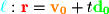)